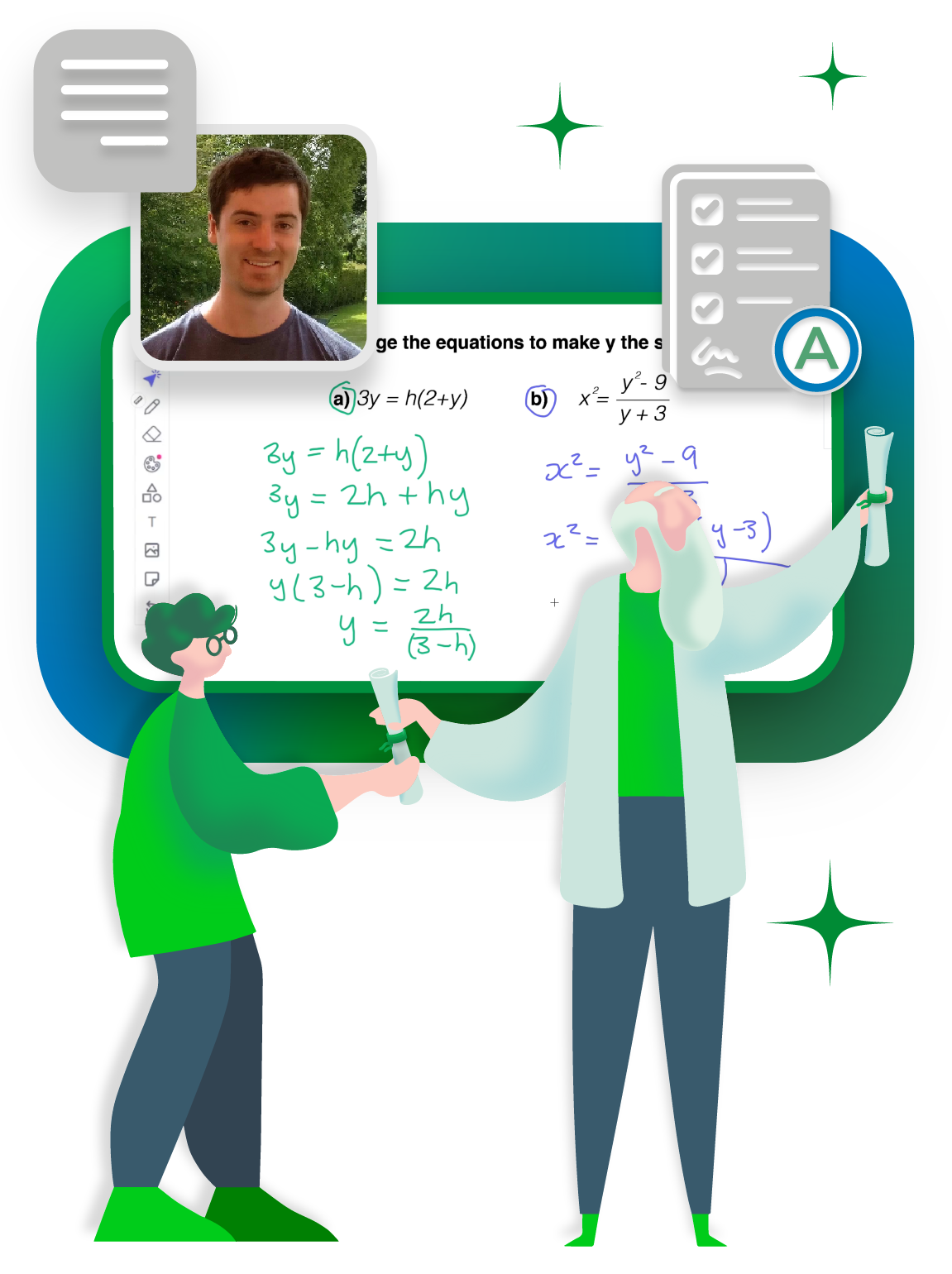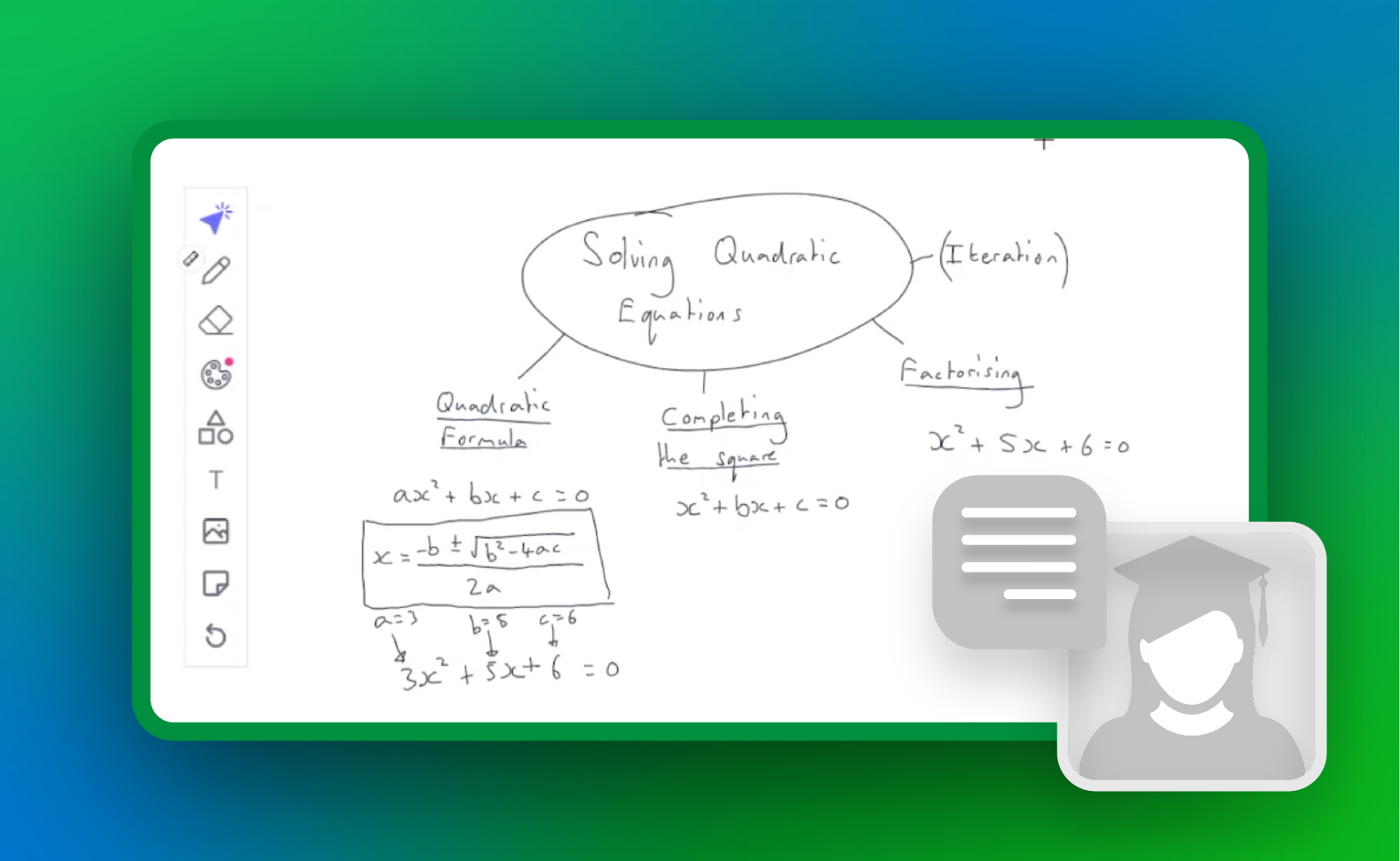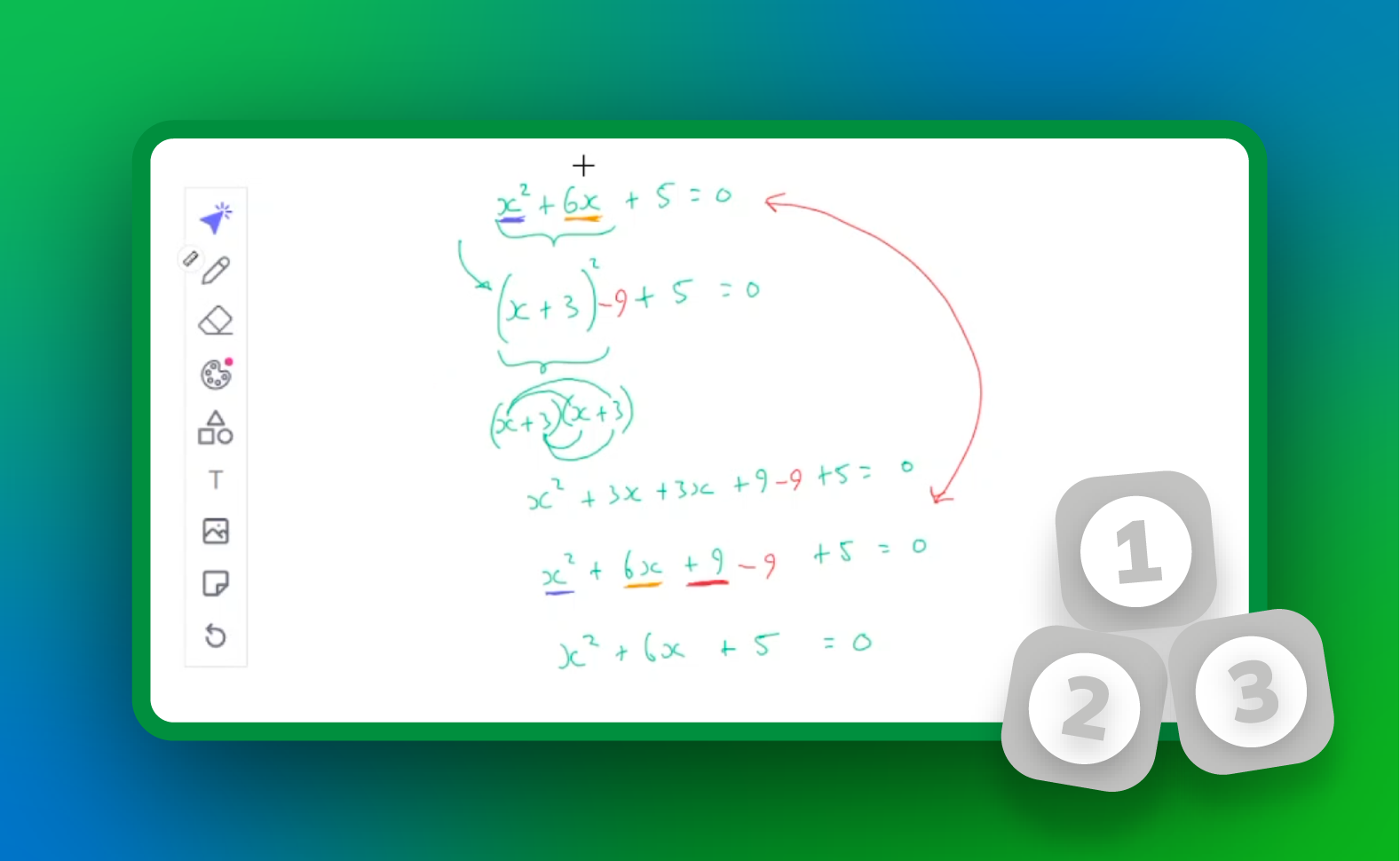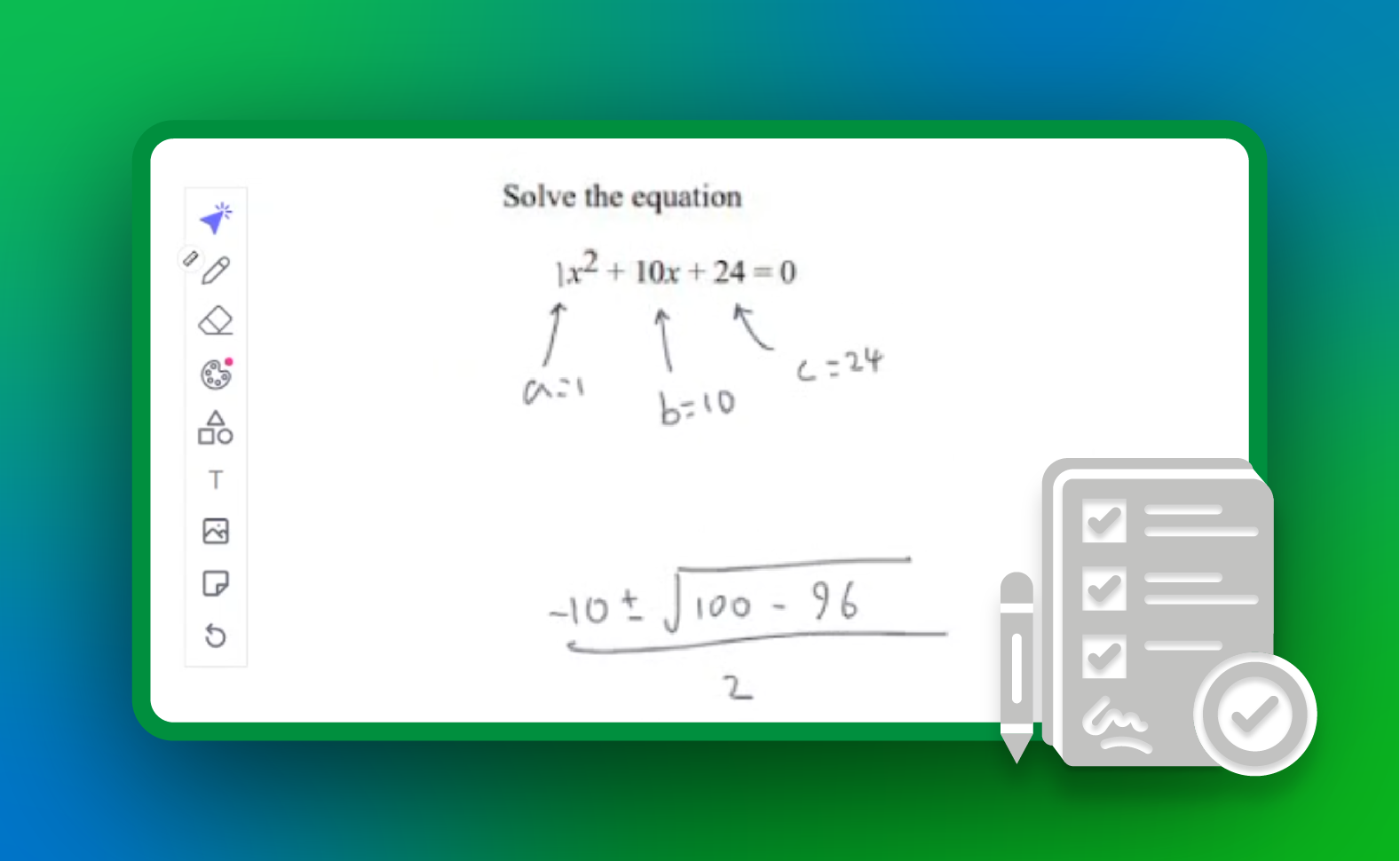Sherpa## 01 Syllabus Overview

Get a look at all of the content covered in the course.

Our free GCSE Maths course is comprised of 46 easy to understand, engaging videos designed to teach you the syllabus with no unnecessary filler.

1. ### Number

1. Whole Numbers 10:24
2. Decimals 12:07
3. Converting fractions, decimals and percentages 10:15
4. Approximation 10:07
5. Fractions 10:03
6. Multiples and factors 14:03
7. Laws of indices 12:30
8. Standard form 12:53
9. Surds 12:49
10. Financial Maths 13:09
2. ### Algebra

1. Algebraic expressions 13:26
2. Algebraic formulae 12:39
3. Linear equations 11:04
4. Simultaneous equations 12:58
6. Inequalities 10:11
7. Sequences 12:12
8. Straight line graphs 13:25
9. Graphs 11:36
10. Transformation of curves 12:49
11. Algebraic fractions 12:29
12. Using and interpreting graphs 12:16
3. See all topics

## 02 How it works

Fun, educational videos from subject experts.

Here's how each topic is broken down:

1.### Introduction

What makes this topic interesting and why it's used.

2.### Explanation

A clear and concise explanation of the topic.

3.### Practice Question

A chance to apply your knowledge and understand a worked example.

"These videos are so helpful - they let me understand the content in my own time and have made me more confident for my mock exams."David Philpott
GCSE Student